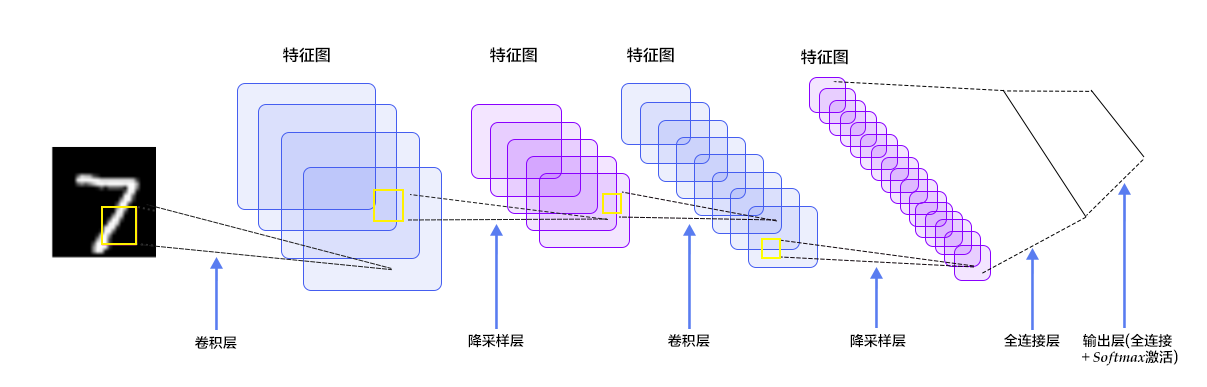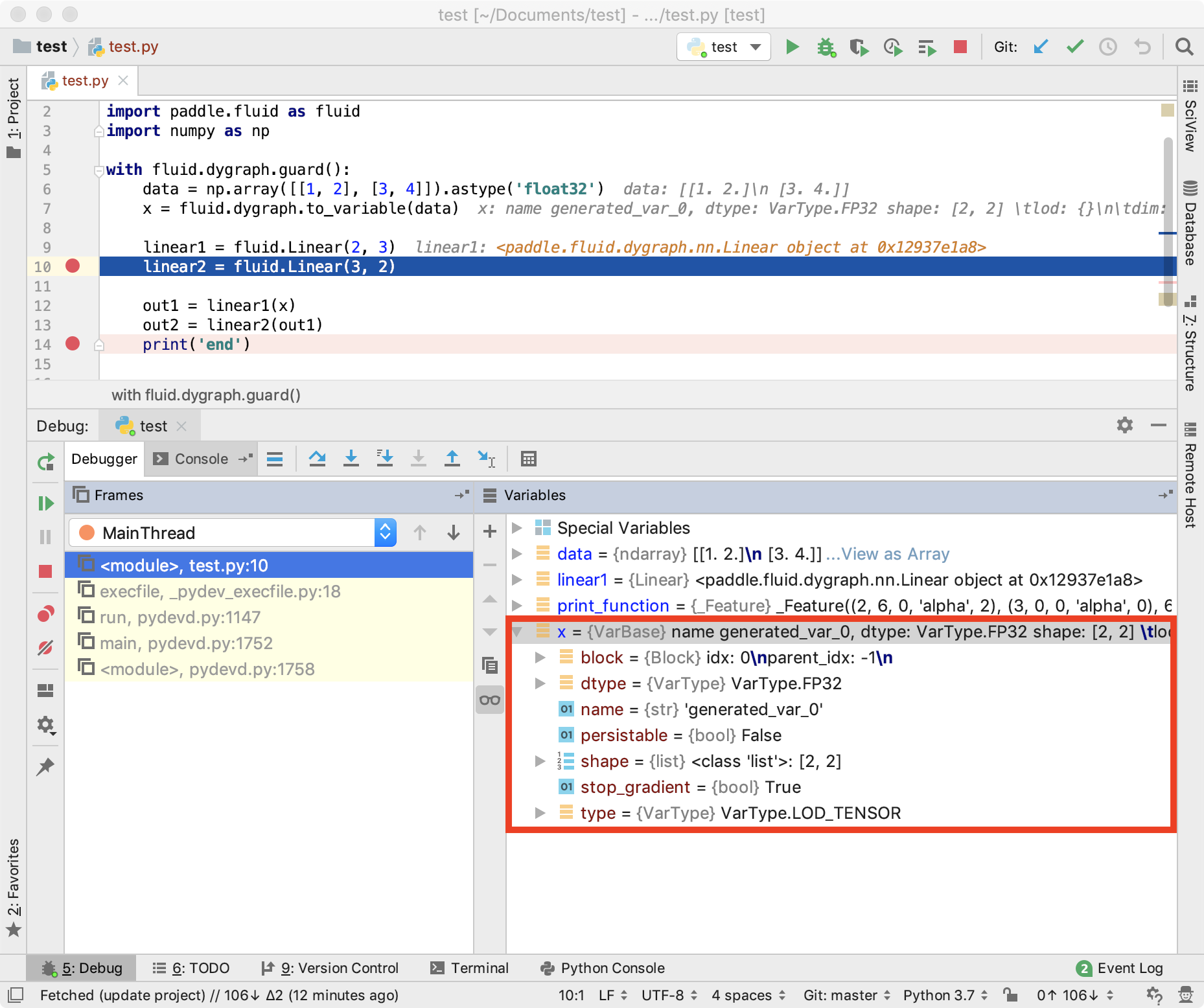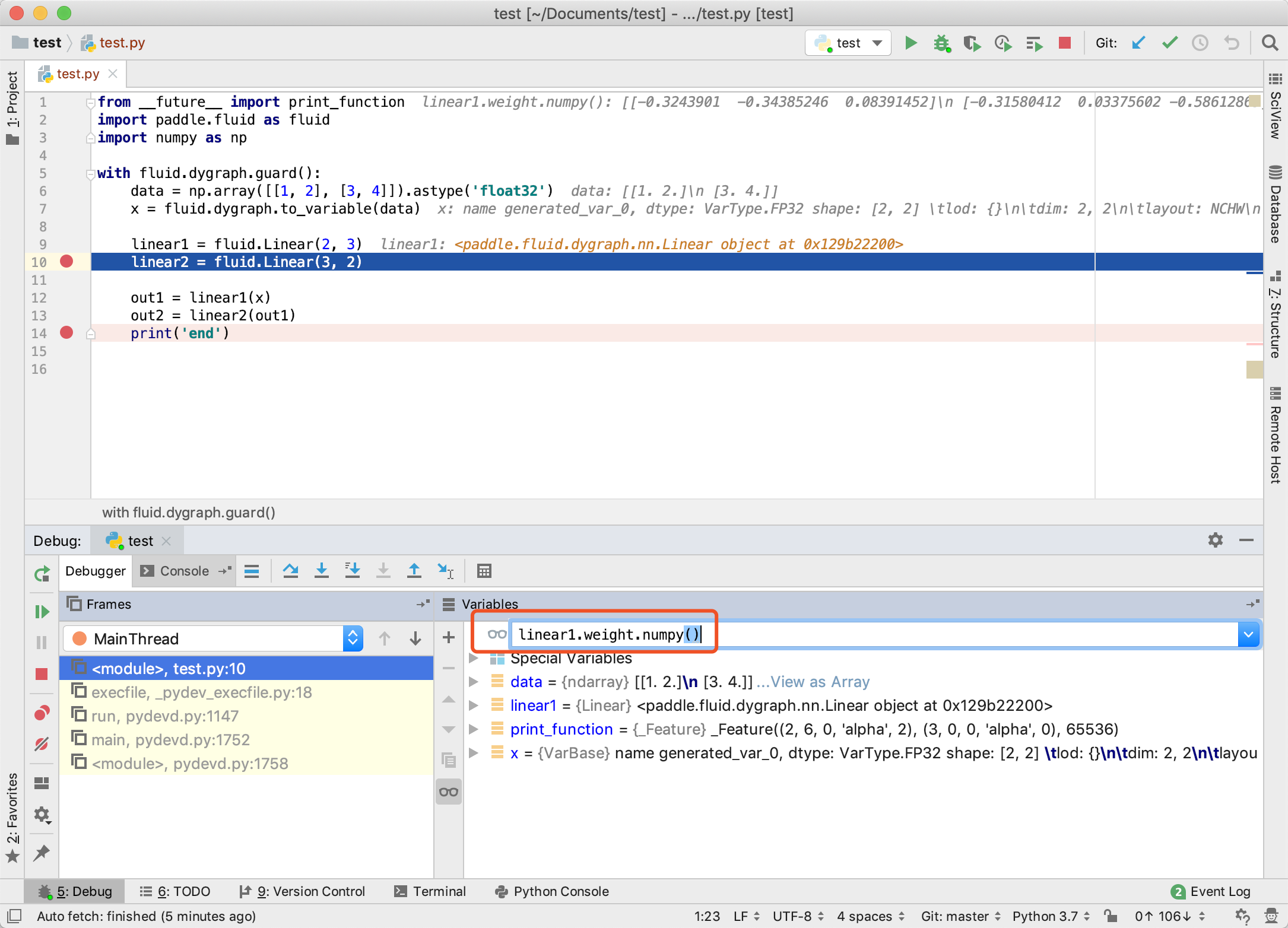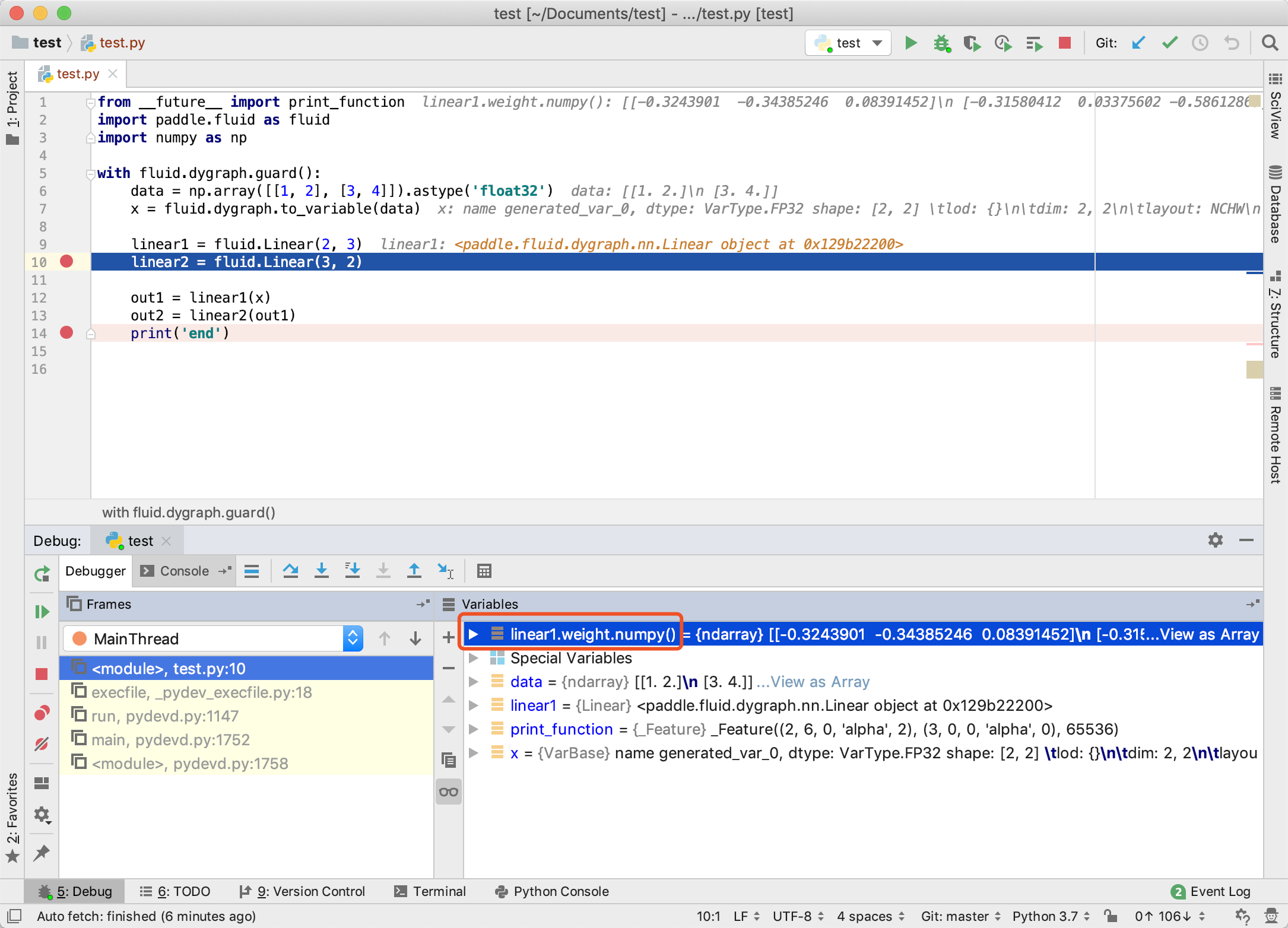# 命令式编程使用教程¶## 为什么命令式编程模式越来越流行？¶

1. 采用先编译后执行的方式，组网阶段和执行阶段割裂，导致调试不方便。
2. 属于一种符号化的编程方式，要学习新的编程方式，有一定的入门门槛。
3. 网络结构固定，对于一些树结构的任务支持的不够好。

1. 代码运行完成后，可以立马获取结果，支持使用 IDE 断点调试功能，使得调试更方便。
2. 属于命令式的编程方式，与编写Python的方式类似，更容易上手。
3. 网络的结构在不同的层次中可以变化，使用更灵活。

1. 如何开启命令式编程模式
2. 如何使用命令式编程进行模型训练
3. 如何基于命令式编程进行多卡训练
4. 如何部署命令式编程模型
5. 命令式编程模式常见的使用技巧，如中间变量值/梯度打印、断点调试、阻断反向传递，以及某些场景下如何改写为静态图模式运行。

## 1. 开启命令式编程模式¶

```with fluid.dygraph.guard()
```

```import numpy as np

main_program = fluid.Program()
startup_program = fluid.Program()
with fluid.program_guard(main_program=main_program, startup_program=startup_program):
# 利用np.ones函数构造出[2*2]的二维数组，值为1
data = np.ones([2, 2], np.float32)

# 静态图模式下，使用layers.data构建占位符用于数据输入
x = fluid.layers.data(name='x', shape=, dtype='float32')
print('In static mode, after calling layers.data, x = ', x)
# 静态图模式下，对Variable类型的数据执行x=x+10操作
x += 10
# 在静态图模式下，需要用户显示指定运行设备
# 此处调用fluid.CPUPlace() API来指定在CPU设备上运行程序
place = fluid.CPUPlace()
# 创建“执行器”，并用place参数指明需要在何种设备上运行
exe = fluid.Executor(place=place)
# 初始化操作，包括为所有变量分配空间等，比如上面的‘x’，在下面这行代码执行后才会被分配实际的内存空间
exe.run(fluid.default_startup_program())
# 使用执行器“执行”已经记录的所有操作，在本例中即执行layers.data、x += 10操作
# 在调用执行器的run接口时，可以通过fetch_list参数来指定要获取哪些变量的计算结果，这里我们要获取‘x += 10’执行完成后‘x’的结果；
# 同时也可以通过feed参数来传入数据，这里我们将data数据传递给‘fluid.layers.data’指定的‘x’。
data_after_run = exe.run(fetch_list=[x], feed={'x': data})
# 此时我们打印执行器返回的结果，可以看到“执行”后，Tensor中的数据已经被赋值并进行了运算，每个元素的值都是11
print('In static mode, data after run:', data_after_run)

# 开启动态图模式
with fluid.dygraph.guard():
# 动态图模式下，将numpy的ndarray类型的数据转换为Variable类型
x = fluid.dygraph.to_variable(data)
print('In DyGraph mode, after calling dygraph.to_variable, x = ', x)
# 动态图模式下，对Variable类型的数据执行x=x+10操作
x += 10
# 动态图模式下，调用Variable的numpy函数将Variable类型的数据转换为numpy的ndarray类型的数据
print('In DyGraph mode, data after run:', x.numpy())
```
```In static mode, after calling layers.data, x =  name: "x"
type {
type: LOD_TENSOR
lod_tensor {
tensor {
data_type: FP32
dims: -1
dims: 2
}
lod_level: 0
}
}
persistable: false

In static mode, data after run: [array([[11., 11.],
[11., 11.]], dtype=float32)]
In DyGraph mode, after calling dygraph.to_variable, x =  name generated_var_0, dtype: VarType.FP32 shape: [2, 2] 	lod: {}
dim: 2, 2
layout: NCHW
dtype: float
data: [1 1 1 1]

In DyGraph mode, data after run: [[11. 11.]
[11. 11.]]

/opt/conda/envs/python35-paddle120-env/lib/python3.7/site-packages/paddle/fluid/executor.py:804: UserWarning: There are no operators in the program to be executed. If you pass Program manually, please use fluid.program_guard to ensure the current Program is being used.
warnings.warn(error_info)
```

• 动态图模式下，所有操作在运行时就已经完成，更接近我们平时的编程方式，可以随时获取每一个操作的执行结果。
• 静态图模式下，过程中并没有实际执行操作，上述例子中可以看到只能打印声明的类型，最后需要调用执行器来统一执行所有操作，计算结果需要通过执行器统一返回。

## 2. 使用命令式编程进行模型训练¶

• 2.1 定义数据读取器：读取数据和预处理操作。
• 2.2 定义模型和优化器：搭建神经网络结构。
• 2.3 训练：配置优化器、学习率、训练参数。循环调用训练过程，循环执行“前向计算 + 损失函数 + 反向传播”。
• 2.4 评估测试：将训练好的模型保存并评估测试。

• 2.5 模型参数的保存和加载方法。

### 2.1 定义数据读取器¶

```import paddle

# 定义批大小
BATCH_SIZE = 64

```
```Cache file /home/aistudio/.cache/paddle/dataset/mnist/train-images-idx3-ubyte.gz not found, downloading https://dataset.bj.bcebos.com/mnist/train-images-idx3-ubyte.gz

........

..
```

### 2.2 定义模型和优化器¶```import paddle.fluid as fluid

# 定义SimpleImgConvPool网络，必须继承自fluid.dygraph.Layer
# 该网络由一个卷积层和一个池化层组成
class SimpleImgConvPool(fluid.dygraph.Layer):
# 在__init__构造函数中会执行变量的初始化、参数初始化、子网络初始化的操作
# 本例中执行了Conv2D和Pool2D网络的初始化操作
def __init__(self,
num_channels,
num_filters,
filter_size,
pool_size,
pool_stride,
pool_type='max',
global_pooling=False,
conv_stride=1,
conv_dilation=1,
conv_groups=1,
act=None,
use_cudnn=False,
param_attr=None,
bias_attr=None):
super(SimpleImgConvPool, self).__init__()

# Conv2D网络的初始化
self._conv2d = Conv2D(
num_channels=num_channels,
num_filters=num_filters,
filter_size=filter_size,
stride=conv_stride,
dilation=conv_dilation,
groups=conv_groups,
param_attr=None,
bias_attr=None,
act=act,
use_cudnn=use_cudnn)

# Pool2D网络的初始化
self._pool2d = Pool2D(
pool_size=pool_size,
pool_type=pool_type,
pool_stride=pool_stride,
global_pooling=global_pooling,
use_cudnn=use_cudnn)

# forward函数实现了SimpleImgConvPool网络的执行逻辑
def forward(self, inputs):
x = self._conv2d(inputs)
x = self._pool2d(x)
return x
```

1. 定义 __init__ 构造函数。

1. 定义 forward 函数。

```# 定义MNIST网络，必须继承自fluid.dygraph.Layer
# 该网络由两个SimpleImgConvPool子网络、reshape层、matmul层、softmax层、accuracy层组成
class MNIST(fluid.dygraph.Layer):
# 在__init__构造函数中会执行变量的初始化、参数初始化、子网络初始化的操作
# 本例中执行了self.pool_2_shape变量、matmul层中参数self.output_weight、SimpleImgConvPool子网络的初始化操作
def __init__(self):
super(MNIST, self).__init__()
self._simple_img_conv_pool_1 = SimpleImgConvPool(
1, 20, 5, 2, 2, act="relu")
self._simple_img_conv_pool_2 = SimpleImgConvPool(
20, 50, 5, 2, 2, act="relu")

# self.pool_2_shape变量定义了经过self._simple_img_conv_pool_2层之后的数据
# 除了batch_size维度之外其他维度的乘积
self.pool_2_shape = 50 * 4 * 4
# self.pool_2_shape、SIZE定义了self.output_weight参数的维度
SIZE = 10
# 定义全连接层的参数
self.output_weight = self.create_parameter(
[self.pool_2_shape, 10])

# forward函数实现了MNIST网络的执行逻辑
def forward(self, inputs, label=None):
x = self._simple_img_conv_pool_1(inputs)
x = self._simple_img_conv_pool_2(x)
x = fluid.layers.reshape(x, shape=[-1, self.pool_2_shape])
x = fluid.layers.matmul(x, self.output_weight)
x = fluid.layers.softmax(x)
if label is not None:
acc = fluid.layers.accuracy(input=x, label=label)
return x, acc
else:
return x
```

1. 变量的初始化：self.pool_2_shape = 50 * 4 * 4
2. 全连接层参数的创建，通过调用 Layercreate_parameter 接口：self.output_weight = self.create_parameter( [ self.pool_2_shape, 10])
3. 子 Layer 的构造：self._simple_img_conv_pool_1、self._simple_img_conv_pool_2

forward 函数的实现和 前面SimpleImgConvPool 类中的实现方式类似。

```import numpy as np

with fluid.dygraph.guard():
# 定义MNIST类的对象
mnist = MNIST()
# 注意动态图模式下必须传入parameter_list参数，该参数为需要优化的网络参数，本例需要优化mnist网络中的所有参数
```

### 2.3 训练¶

• 数据读取：读取每批数据，通过 to_variable 接口将 numpy.ndarray 对象转换为 Variable 类型的对象。
• 网络正向执行：在正向执行时，用户构造出img和label之后，可利用类似函数调用的方式（如：mnist(img, label)）传递参数执行对应网络的 forward 函数。
• 计算损失值：根据网络返回的计算结果，计算损失值，便于后续执行反向计算。
• 执行反向计算：需要用户主动调用 backward 接口来执行反向计算。
• 参数更新：调用优化器的 minimize 接口对参数进行更新。
• 梯度重置：将本次计算的梯度值清零，以便进行下一次迭代和梯度更新。
• 保存训练好的模型：通过 Layerstate_dict 获取模型的参数；通过 save_dygraph 对模型参数进行保存。
```import numpy as np

with fluid.dygraph.guard():
# 定义MNIST类的对象
mnist = MNIST()
# 注意动态图模式下必须传入parameter_list参数，该参数为需要优化的网络参数，本例需要优化mnist网络中的所有参数

# 设置全部样本的训练次数
epoch_num = 5

# 执行epoch_num次训练
for epoch in range(epoch_num):
# 读取训练数据进行训练
dy_x_data = np.array([x.reshape(1, 28, 28) for x in data]).astype('float32')
y_data = np.array([x for x in data]).astype('int64').reshape(-1, 1)

#将ndarray类型的数据转换为Variable类型
img = to_variable(dy_x_data)
label = to_variable(y_data)

# 网络正向执行
cost, acc = mnist(img, label)

# 计算损失值
loss = fluid.layers.cross_entropy(cost, label)
avg_loss = fluid.layers.mean(loss)
# 执行反向计算
avg_loss.backward()
# 参数更新
# 将本次计算的梯度值清零，以便进行下一次迭代和梯度更新

# 输出对应epoch、batch_id下的损失值
if batch_id % 100 == 0:
print("Loss at epoch {} step {}: {:}".format(
epoch, batch_id, avg_loss.numpy()))

# 保存训练好的模型
model_dict = mnist.state_dict()
fluid.save_dygraph(model_dict, "save_temp")
```
```Loss at epoch 0 step 0: [3.362183]
Loss at epoch 0 step 100: [0.20108832]
Loss at epoch 0 step 200: [0.1681692]
Loss at epoch 0 step 300: [0.11894853]
Loss at epoch 0 step 400: [0.13005154]
Loss at epoch 0 step 500: [0.10004535]
Loss at epoch 0 step 600: [0.11465541]
Loss at epoch 0 step 700: [0.14584845]
Loss at epoch 0 step 800: [0.21515566]
Loss at epoch 0 step 900: [0.13847716]
Loss at epoch 1 step 0: [0.03004131]
Loss at epoch 1 step 100: [0.1855965]
Loss at epoch 1 step 200: [0.07302501]
Loss at epoch 1 step 300: [0.02016284]
Loss at epoch 1 step 400: [0.03899964]
Loss at epoch 1 step 500: [0.05415711]
Loss at epoch 1 step 600: [0.09633664]
Loss at epoch 1 step 700: [0.07155745]
Loss at epoch 1 step 800: [0.13023862]
Loss at epoch 1 step 900: [0.09051394]
Loss at epoch 2 step 0: [0.00580437]
Loss at epoch 2 step 100: [0.1506507]
Loss at epoch 2 step 200: [0.03713503]
Loss at epoch 2 step 300: [0.01145383]
Loss at epoch 2 step 400: [0.0497771]
```

### 2.4 评估测试¶

```model.eval()      #切换到评估模式
model.train()     #切换到训练模式
```

• 首先定义 MNIST 类的对象 mnist_eval，然后通过 load_dygraph 接口加载保存好的模型参数，通过 Layerset_dict 接口将参数导入到模型中，通过 Layer 的 eval 接口切换到预测评估模式。
• 读取测试数据执行网络正向计算，进行评估测试，输出不同 batch 数据下损失值和准确率的平均值。
```with fluid.dygraph.guard():
# 定义MNIST类的对象
mnist_eval = MNIST()

# 加载保存的模型
mnist_eval.set_dict(model_dict)

# 切换到预测评估模式
mnist_eval.eval()

acc_set = []
avg_loss_set = []
# 读取测试数据进行评估测试
dy_x_data = np.array([x.reshape(1, 28, 28)
for x in data]).astype('float32')
y_data = np.array(
[x for x in data]).astype('int64').reshape(-1, 1)

# 将ndarray类型的数据转换为Variable类型
img = to_variable(dy_x_data)
label = to_variable(y_data)

# 网络正向执行
prediction, acc = mnist_eval(img, label)

# 计算损失值
loss = fluid.layers.cross_entropy(input=prediction, label=label)
avg_loss = fluid.layers.mean(loss)
acc_set.append(float(acc.numpy()))
avg_loss_set.append(float(avg_loss.numpy()))

# 输出不同 batch 数据下损失值和准确率的平均值
acc_val_mean = np.array(acc_set).mean()
avg_loss_val_mean = np.array(avg_loss_set).mean()
print("Eval avg_loss is: {}, acc is: {}".format(avg_loss_val_mean, acc_val_mean))
```

### 2.5 模型参数的保存和加载¶

1. 保存模型参数：首先通过 minist.state_dict 函数获取 mnist 网络的所有参数，然后通过 fluid.save_dygraph 函数将获得的参数保存至以 save_path 为前缀的文件中。
• Layerstate_dict 接口：该接口可以获取当前层及其子层的所有参数，并将参数存放在 dict 结构中。
• Optimizer 的 state_dict 接口：该接口可以获取优化器的信息，并将信息存放在 dict 结构中。其中包含优化器使用的所有变量，例如对于 Adam 优化器，包括 beta1、beta2、momentum 等信息。注意如果该优化器的 minimize 函数没有被调用过，则优化器的信息为空。
• save_dygraph 接口：该接口将传入的参数或优化器的 dict 保存到磁盘上。
```# 保存模型参数
1. fluid.save_dygraph(minist.state_dict(), “save_path”)
# 保存优化器信息
```

1. 通过 fluid.load_dygraph 函数获取模型参数信息 model_state 和优化器信息 opt_state；
2. 通过 mnist.set_dict 函数用获取的模型参数信息设置 mnist 网络的参数
• Layerset_dict 接口：该接口根据传入的 dict 结构设置参数，所有参数将由 dict 结构中的 Tensor 设置。
• Optimizer 的 set_dict 接口：该接口根据传入的 dict 结构设置优化器信息，例如对于 Adam 优化器，包括 beta1、beta2、momentum 等信息。如果使用了 LearningRateDecay ，则 global_step 信息也将会被设置。
```# 获取模型参数和优化器信息
# 加载模型参数
2. mnist.set_dict(model_state)
# 加载优化器信息
```

## 3. 多卡训练¶

1. 本实例仍然采用前面定义的 MNIST 网络，可将前面定义的 SimpleImgConvPool、MNIST 网络结构、相关的库导入代码、以及下面多卡训练的示例代码拷贝至本地文件 train.py 中。
```import numpy as np

# 通过 Env() 的 dev_id 设置程序运行的设备
place = fluid.CUDAPlace(fluid.dygraph.parallel.Env().dev_id)
with fluid.dygraph.guard(place):
# 准备多卡环境
strategy = fluid.dygraph.parallel.prepare_context()
epoch_num = 5
BATCH_SIZE = 64
mnist = MNIST()
# 数据并行模块
mnist = fluid.dygraph.parallel.DataParallel(mnist, strategy)

# 数据切分

for epoch in range(epoch_num):
dy_x_data = np.array([x.reshape(1, 28, 28)
for x in data]).astype('float32')
y_data = np.array(
[x for x in data]).astype('int64').reshape(-1, 1)

img = fluid.dygraph.to_variable(dy_x_data)
label = fluid.dygraph.to_variable(y_data)

# 网络正向执行
cost, acc = mnist(img, label)

# 计算损失值
loss = fluid.layers.cross_entropy(cost, label)
avg_loss = fluid.layers.mean(loss)

# 单步训练：首先对 loss 进行归一化，然后计算单卡的梯度，最终将所有的梯度聚合
avg_loss = mnist.scale_loss(avg_loss)
avg_loss.backward()

# 参数更新
# 将本次计算的梯度值清零，以便进行下一次迭代和梯度更新

# 输出对应epoch、batch_id下的损失值
if batch_id % 100 == 0 and batch_id is not 0:
print("epoch: {}, batch_id: {}, loss is: {}".format(epoch, batch_id, avg_loss.numpy()))
```

2、飞桨动态图多进程多卡模型训练启动时，需要指定使用的 GPU，比如使用 0,1 卡，可执行如下命令启动训练：

```CUDA_VISIBLE_DEVICES=0,1 python -m paddle.distributed.launch --log_dir ./mylog train.py
```

```-----------  Configuration Arguments -----------
cluster_node_ips: 127.0.0.1
log_dir: ./mylog
node_ip: 127.0.0.1
print_config: True
selected_gpus: 0,1
started_port: 6170
training_script: train.py
training_script_args: []
------------------------------------------------
trainers_endpoints: 127.0.0.1:6170,127.0.0.1:6171 , node_id: 0 , current_node_ip: 127.0.0.1 , num_nodes: 1 , node_ips: ['127.0.0.1'] , nranks: 2
```

```.
├── mylog
│   ├── workerlog.0
│   └── workerlog.1
└── train.py
```

1. 通过 Env() 的 dev_id 设置程序运行的设备。
```place = fluid.CUDAPlace(fluid.dygraph.parallel.Env().dev_id)
with fluid.dygraph.guard(place):
```
1. 准备多卡环境。
```strategy = fluid.dygraph.parallel.prepare_context()
```
1. 数据并行模块。

```mnist = fluid.dygraph.parallel.DataParallel(mnist, strategy)
```
1. 数据切分。

```train_reader = fluid.contrib.reader.distributed_batch_reader(train_reader)
```
1. 单步训练。

```avg_loss = mnist.scale_loss(avg_loss)
avg_loss.backward()
```
1. 模型保存。

```if fluid.dygraph.parallel.Env().local_rank == 0：
fluid.save_dygraph(mnist.state_dict, “path”)
```
1. 评估测试。

## 4. 模型部署¶

• 如果用户使用的 if/else、switch、for/while 与输入（包括输入的值和 shape ）无关，则可以使用如下动态图模型部署方案：
• 使用 TracedLayer 将前向动态图模型转换为静态图模型。可以将动态图保存后做在线C++预测；除此以外，用户也可使用转换后的静态图模型在Python端做预测，通常比原先的动态图性能更好。
• 所有的TracedLayer对象均不应通过构造函数创建，而需通过调用静态方法 TracedLayer.trace(layer, inputs) 创建。
• TracedLayer使用 Executor 和 CompiledProgram 运行静态图模型。
```from paddle.fluid.dygraph import TracedLayer

with fluid.dygraph.guard():
# 定义MNIST类的对象
mnist = MNIST()
in_np = np.random.random([10, 1, 28, 28]).astype('float32')
# 将numpy的ndarray类型的数据转换为Variable类型
input_var = fluid.dygraph.to_variable(in_np)
# 通过 TracerLayer.trace 接口将动态图模型转换为静态图模型
out_dygraph, static_layer = TracedLayer.trace(mnist, inputs=[input_var])
save_dirname = './saved_infer_model'
# 将转换后的模型保存
static_layer.save_inference_model(save_dirname, feed=, fetch=)
```
```# 静态图中需要使用执行器执行之前已经定义好的网络
place = fluid.CPUPlace()
exe = fluid.Executor(place)
program, feed_vars, fetch_vars = fluid.io.load_inference_model(save_dirname, exe)
# 静态图中需要调用执行器的run方法执行计算过程
fetch, = exe.run(program, feed={feed_vars: in_np}, fetch_list=fetch_vars)
```

save_inference_model 保存的下来的模型，同样可以使用 C++ 加载部署，具体的操作请参考：C++ 预测 API介绍

• 如果任务中包含了依赖数据的控制流，比如下面这个示例中if条件的判断依赖输入的shape。针对这种场景，可以使用基于ProgramTranslator的方式转成静态图的program，通过save_inference_model 接口将静态图模型保存为用于预测部署的模型，之后利用 load_inference_model 接口将保存的模型加载，并使用 Executor 执行，检查结果是否正确。

```with fluid.dygraph.guard():
in_np = np.array([-2]).astype('int')
# 将numpy的ndarray类型的数据转换为Variable类型
input_var = fluid.dygraph.to_variable(in_np)
# if判断与输入input_var的shape有关
if input_var.shape > 1:
print("input_var's shape > 1")
else:
print("input_var's shape < 1")
```
• 针对依赖数据的控制流，解决流程如下 1. 添加declarative装饰器； 2. 利用ProgramTranslator进行转换
1. 添加declarative装饰器 首先需要对给MNist类的forward函数添加一个declarative 装饰器，来标记需要转换的代码块，（注：需要在最外层的class的forward函数中添加）
```from paddle.fluid.dygraph.jit import declarative

# 定义MNIST网络，必须继承自fluid.dygraph.Layer
# 该网络由两个SimpleImgConvPool子网络、reshape层、matmul层、softmax层、accuracy层组成
class MNIST(fluid.dygraph.Layer):
# 在__init__构造函数中会执行变量的初始化、参数初始化、子网络初始化的操作
# 本例中执行了self.pool_2_shape变量、matmul层中参数self.output_weight、SimpleImgConvPool子网络的初始化操作
def __init__(self):
super(MNIST, self).__init__()
self._simple_img_conv_pool_1 = SimpleImgConvPool(
1, 20, 5, 2, 2, act="relu")
self._simple_img_conv_pool_2 = SimpleImgConvPool(
20, 50, 5, 2, 2, act="relu")

# self.pool_2_shape变量定义了经过self._simple_img_conv_pool_2层之后的数据
# 除了batch_size维度之外其他维度的乘积
self.pool_2_shape = 50 * 4 * 4
# self.pool_2_shape、SIZE定义了self.output_weight参数的维度
SIZE = 10
# 定义全连接层的参数
self.output_weight = self.create_parameter(
[self.pool_2_shape, 10])

# forward函数实现了MNIST网络的执行逻辑
@declarative
def forward(self, inputs, label=None):
x = self._simple_img_conv_pool_1(inputs)
x = self._simple_img_conv_pool_2(x)
x = fluid.layers.reshape(x, shape=[-1, self.pool_2_shape])
x = fluid.layers.matmul(x, self.output_weight)
x = fluid.layers.softmax(x)
if label is not None:
acc = fluid.layers.accuracy(input=x, label=label)
return x, acc
else:
return x
```
```  File "<ipython-input-1-b7b25c28bae2>", line 25
@declarative
^
TabError: inconsistent use of tabs and spaces in indentation
```

2） 利用ProgramTranslator进行转换

```from paddle.fluid.dygraph.dygraph_to_static import ProgramTranslator

with fluid.dygraph.guard():
prog_trans = fluid.dygraph.ProgramTranslator()
mnist = MNIST()

in_np = np.random.random([10, 1, 28, 28]).astype('float32')
label_np = np.random.randint(0, 10, size=(10,1)).astype( "int64")
input_var = fluid.dygraph.to_variable(in_np)
label_var = flui.dyraph.to_variable(label_np)

out = mnist( input_var, label_var)

prog_trans.save_inference_model("./mnist_dy2stat", fetch=[0,1])
```

## 5. 使用技巧¶

### 5.1 中间变量值、梯度打印¶

1. 用户想要查看任意变量的值，可以使用 numpy 接口。
```x = y * 10
print(x.numpy())
```

1. 查看反向的值 可以在执行了 backward 之后，可以通过 gradient 接口来看任意变量的梯度
```x = y * 10
x.backward()
```

### 5.2 断点调试¶

1. 如下图所示，在示例程序中设置两个断点，执行到第一个断点的位置，我们可以观察变量 x 和 linear1 的信息。1. 同时可以观察 linear1 中的权重值。### 5.3 使用声明式编程模式运行¶

1. 当用户使用的 if/else、switch、for/while 与输入（包括输入的值和 shape ）无关时，可以在不改动模型定义的情况下使用静态图的模式运行。该方法将模型训练改为了静态图模式，区别于第4小节仅预测部署改为了静态图模式。
1. 如果使用了与输入相关的控制流，请参照如何把动态图转写成静态图章节，将动态图代码进行转写。

```# 设置全部样本训练次数（epoch_num）、批大小（BATCH_SIZE）。
epoch_num = 1
BATCH_SIZE = 64

main_program = fluid.Program()
startup_program = fluid.Program()

with fluid.program_guard(main_program=main_program, startup_program=startup_program):
# 静态图中需要使用执行器执行之前已经定义好的网络
exe = fluid.Executor(fluid.CPUPlace())

# 定义MNIST类的对象，可以使用动态图定义好的网络结构
mnist_static = MNIST()
# 定义优化器对象，静态图模式下不需要传入parameter_list参数
sgd_static = fluid.optimizer.SGDOptimizer(learning_rate=1e-3)

# 静态图需要明确定义输入变量，即“占位符”，在静态图组网阶段并没有读入数据，所以需要使用占位符指明输入数据的类型，shape等信息
img_static = fluid.data(
name='pixel', shape=[None, 1, 28, 28], dtype='float32')
label_static = fluid.data(name='label', shape=[None, 1], dtype='int64')

# 调用网络，执行前向计算
cost_static = mnist_static(img_static)
# 计算损失值
loss_static = fluid.layers.cross_entropy(cost_static, label_static)
avg_loss_static = fluid.layers.mean(loss_static)

# 调用优化器的minimize接口计算和更新梯度
sgd_static.minimize(avg_loss_static)

# 静态图中需要显示对网络进行初始化操作
exe.run(fluid.default_startup_program())

for epoch in range(epoch_num):
x_data_static = np.array(
[x.reshape(1, 28, 28)
for x in data]).astype('float32')
y_data_static = np.array(
[x for x in data]).astype('int64').reshape([BATCH_SIZE, 1])

fetch_list = [avg_loss_static.name]
# 静态图中需要调用执行器的run方法执行计算过程，需要获取的计算结果（如avg_loss）需要通过fetch_list指定
out = exe.run(
fluid.default_main_program(),
feed={"pixel": x_data_static,
"label": y_data_static},
fetch_list=fetch_list)

static_out = out

if batch_id % 100 == 0 and batch_id is not 0:
print("epoch: {}, batch_id: {}, loss: {}".format(epoch, batch_id, static_out))
```

1. 定义占位符
• 利用fluid.data 定义占位符，在静态图表示会在执行器执行时才会提供数据。
1. 组网
• 优化器对象在静态图模式下不需要传入parameter_list参数。
• 将定义的占位符，输入给模型执行正向，然后计算损失值，最后利用优化器将损失值做最小化优化，得到要训练的网络。
1. 执行
• 需要对网络进行初始化操作。
• 需要使用执行器执行之前已经定义好的网络，需要调用执行器的run方法执行计算过程。

### 5.4 阻断反向传递¶

1. detach接口（建议用法） 使用方式如下：
```fw_out = fw_out.detach()
```

detach() 接口会产生一个新的、和当前计算图分离的，但是拥有当前变量内容的临时变量。

```import paddle.fluid as fluid
import numpy as np

with fluid.dygraph.guard():
value0 = np.arange(26).reshape(2, 13).astype("float32")
value1 = np.arange(6).reshape(2, 3).astype("float32")
value2 = np.arange(10).reshape(2, 5).astype("float32")

# 将ndarray类型的数据转换为Variable类型
a = fluid.dygraph.to_variable(value0)
b = fluid.dygraph.to_variable(value1)
c = fluid.dygraph.to_variable(value2)

# 构造fc、fc2层
fc = fluid.Linear(13, 5, dtype="float32")
fc2 = fluid.Linear(3, 3, dtype="float32")

# 对fc、fc2层执行前向计算
out1 = fc(a)
out2 = fc2(b)

# 将不会对out1这部分子图做反向计算
out1 = out1.detach()

out = fluid.layers.concat(input=[out1, out2, c], axis=1)
out.backward()

```

```fw_out.stop_gradient = True
```

```import paddle.fluid as fluid
import numpy as np

with fluid.dygraph.guard():
value0 = np.arange(26).reshape(2, 13).astype("float32")
value1 = np.arange(6).reshape(2, 3).astype("float32")
value2 = np.arange(10).reshape(2, 5).astype("float32")

# 将ndarray类型的数据转换为Variable类型
a = fluid.dygraph.to_variable(value0)
b = fluid.dygraph.to_variable(value1)
c = fluid.dygraph.to_variable(value2)

# 构造fc、fc2层
fc = fluid.Linear(13, 5, dtype="float32")
fc2 = fluid.Linear(3, 3, dtype="float32")

# 对fc、fc2层执行前向计算
out1 = fc(a)
out2 = fc2(b)

# 相当于不会对out1这部分子图做反向计算